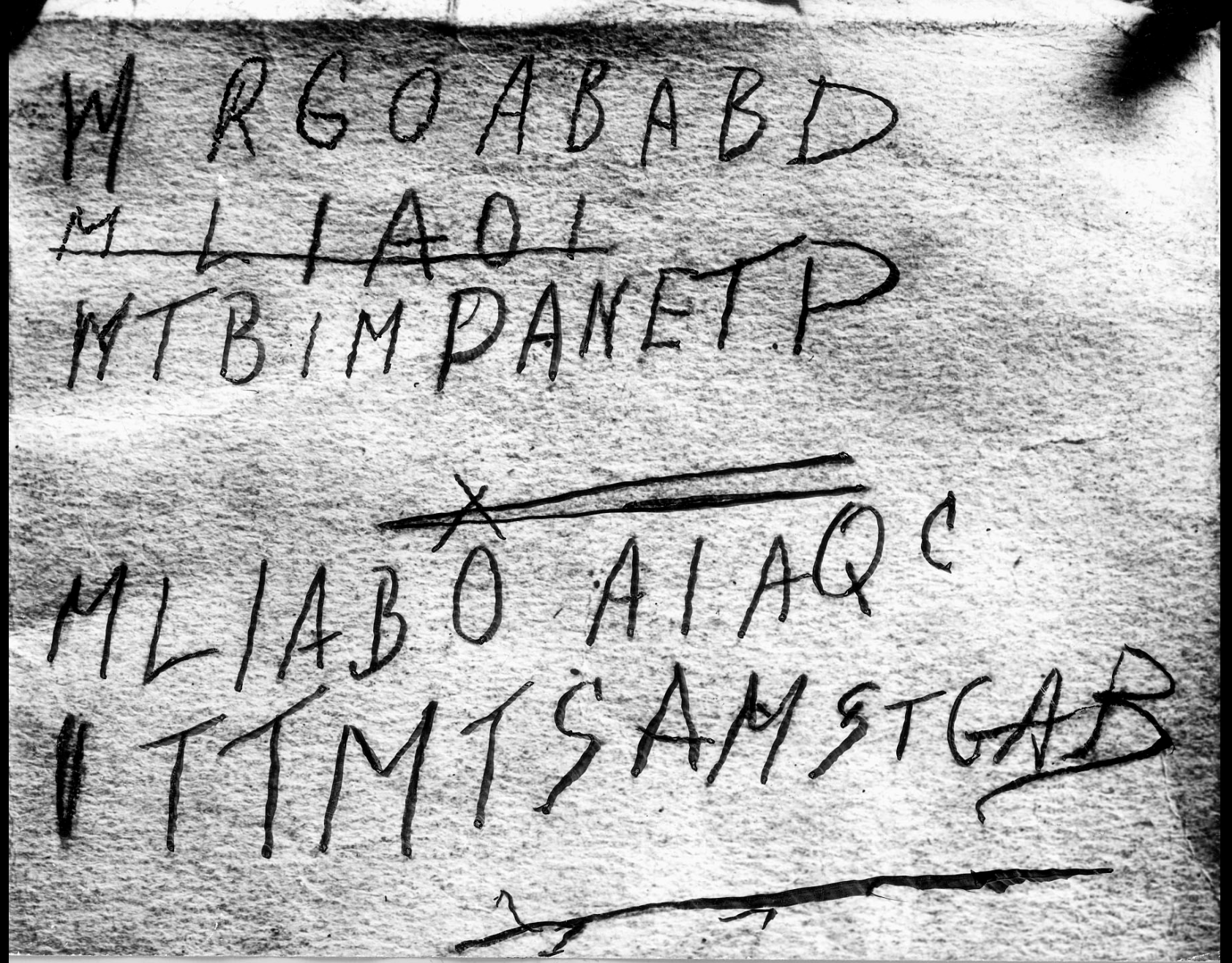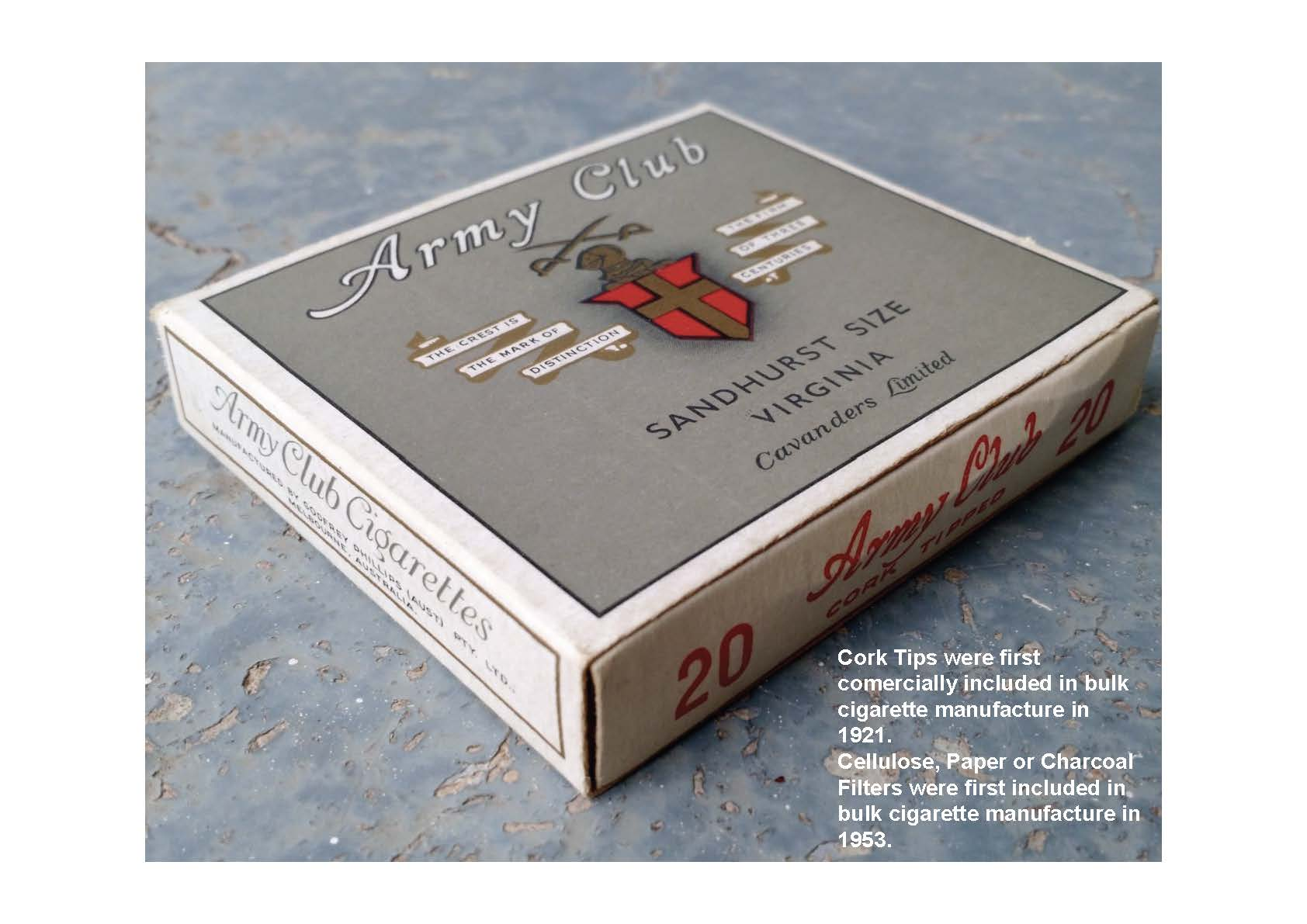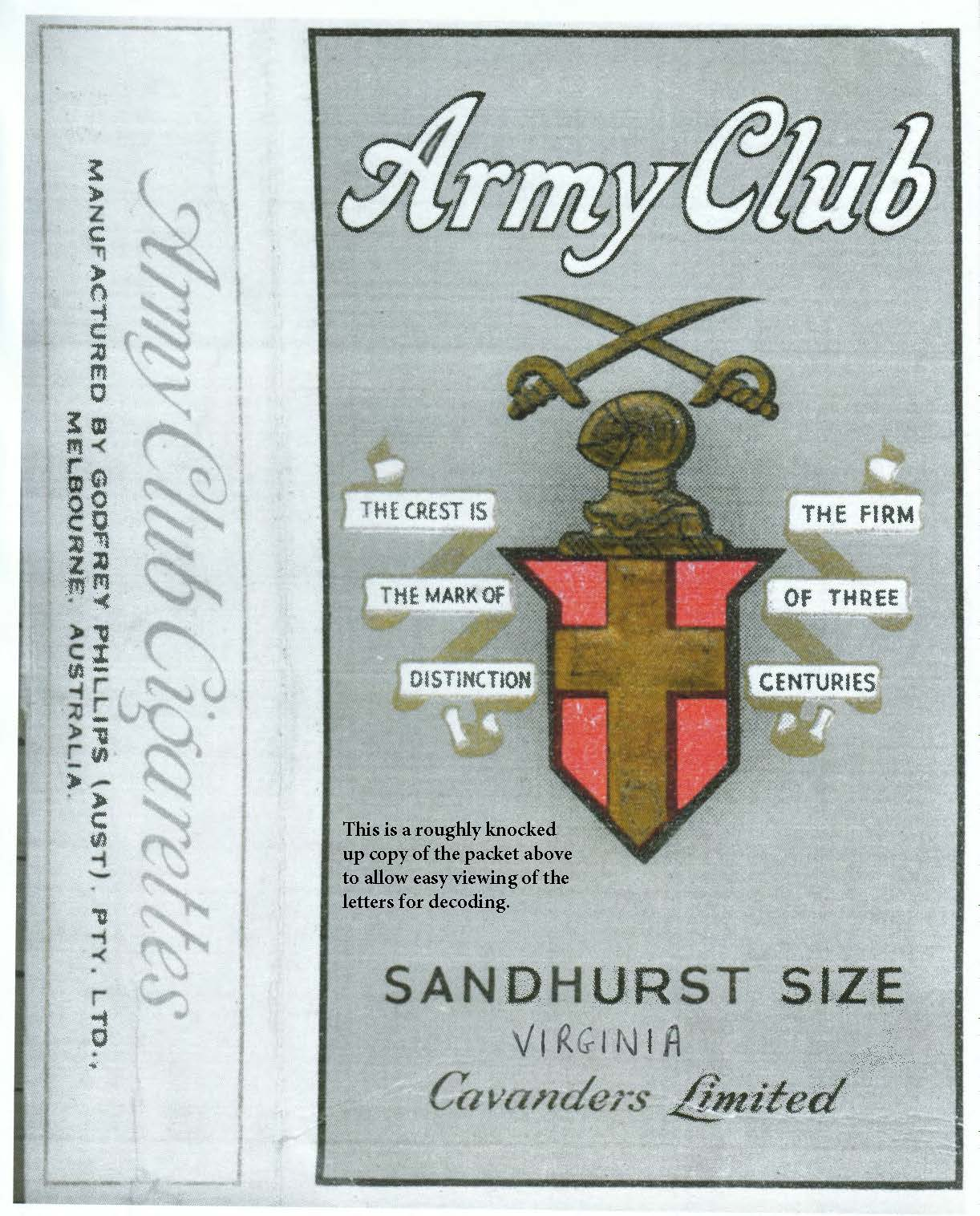# On Somerton Beach – Just letters? Nope! They’re a message! (#4) Tamam Shud

By Alan Hamill

Who is The Somerton Man? Why was he in Adelaide? What was TAMAM SHUD? What happened on Somerton Beach?

The So-Called Laundry Marks!Markings found on the inside lining of a trouser pocket of the Somerton Man. These were referred to as the laundry marks.THE SOMERTON MAN CODE:-Aaaah, the code. Watch how we decode it!This is a copy (above) of the actual Pigeon Code which is yet to be decrypted. This was found in a boarded up fireplace  years after it was sent. The page was still in the red capsule attached to the leg of a pigeon skeleton and is truly a one time pad where the sender burns his page when sent and the receiver burns his page when decrypted.

For an understanding of the functionality of the “One Time Pad” system search for “The Pigeon Code” which was used for message transfer during the “D Day Invasion of Normandy” to free France from German occupation.

To the Code:-

The first two M’s looked from the start as not just M’s but a signal that there was some skipping involved as the middle of these M’s didn’t reach the bottom and what is more important is that they had deliberately been written this way. The later M’s were clearly just M’s for Message.

The 4 lines of the message text are basically 8 letters long with some other letters added before and after, where required, for an action to be done.

As “One Time Pad” codes need both a pad and a key, in this case the pad is the cigarette packet itself, and the key is the “Laundry Marks” in the trousers. i.e. 1171/7 (see the Laundry Marks section above). The 1171 indicates that there would be 7 cigarettes found in any message packet. And the /7 indicates a movement of 7 places.

The only difference here is that the people used this “One Time Pad” code many times, not just the once. This is likely thanks to “George” Marshall for its design.

The first 2 lines of the code use the cigarette packet writing in the top line Army Club, then the block to the left of the shield, then the lower text block.

The second 2 lines of the code use the top line Army Club, then the block to the right of the shield, then the lower text.Godfrey Phillips started making and packaging Army Club Cigarettes under license in Australia in 1930 and the code participants were operating in Australia so an Australian packet was used.
The P is on the side panel. As it’s the only P on the packet it guarantees the only option is an Australian manufactured Army Club pack.

As the number 7 appears after the slash on the laundry marks, you move 7 letter spaces in the direction of the arrow from the originating letter. Military usually went left, right, left, etc.

At the half way point the X and large left arrow means switch to left.

Also of note:- It is unlikely the writer would disguise her name as the sender in/of the code and then write her phone number in normal text mode on the same page, so the phone number had to be written later for some reason.

OK, then let’s break it down!

The methodology is consistent across the whole code, and that is to start at the first occurrence of each code letter on the packet and move in the direction of the arrow.Top Line:-

<-  The first R in the top code line is the second letter in Army. As the arrow points left you go backwards. The r starts at A, then d (in Limited), then e, then t, then i, then m, then the seventh letter to the left is i. So R becomes I.

->  The G in the top code line is the G in Virginia. As the arrow points right you go forwards. The G starts at I, then N, then I, then A, then C in Cavanders, then a, then the seventh letter to the right is v. So G becomes v.

<-  The O in the top code line is the O in “THE MARK OF”. As the arrow points left you go backwards. O starts at K, then R, then A, then M, then E, then H, then the seventh letter to the left is T. So O becomes T.

->  The A in the top code line is the first letter in Army. As the arrow points right you go forwards. A starts at r, then m, then y, then C, then l, then u, then the seventh letter to the right is b. So A becomes B.

<-  The B in the top code line is the b in Club. As the arrow points left you go backwards. The b starts at u, then l, then C, then y, then m, then r, then the seventh letter to the left is A. So B becomes A.

->  The second A in the top code line is the first letter in Army. As the arrow points right you go forwards. A starts at r, then m, then y, then C, then l, then u, then the seventh letter to the right is b. So A becomes B.

<-  The second B in the top code line is the b in Club. As the arrow points left you go backwards. B starts at u, then l, then C, then y, then m, then r, then the seventh letter to the left is A. So B becomes A.

->  The D in the top code line is the first letter N in DISTINCTION. As the arrow points right you go forwards. D starts at I, then S, then T, then I, then N, then C, then the seventh letter to the right is T. So D becomes T.

Second Line:-

T = Transmit

<-  The B in the second code line is the b in Club. As the arrow points left you go backwards. B starts at u, then l, then C, then y, then m, then r, then the seventh letter to the left is A. So B becomes A.

->  The I in the second code line is the I in CREST IS. As the arrow points right you go forwards. I starts at S, then T, then H, then E, then M, then A, then the seventh letter to the right is R. So I becomes R.

<-  The M in the second code line is the third letter in Army. As the arrow points left you go backwards. The m starts at r, then A, then d (in Limited), then e, then t, then i, then the seventh letter to the left is m. So M becomes M.

->  The P in the second code line is the first letter in PHILLIPS on the side panel. As the arrow points right you go forwards. P starts at H, then I, then L, then L, then I, then P, then the seventh letter to the right is S. So P becomes S.

<-  The A in the second code line is the A in Army. As the arrow points left you go backwards. A starts at the d (in Limited), then e, then t, then i, then m, then i, then the seventh letter to the left is L. So A becomes L.

->  The N in the second code line is the first letter N in DISTINCTION. As the arrow points right you go forwards. N starts at C, then T, then I, then O, then N, then S (in SANDHURST), then the seventh letter to the right is A. So N becomes A.

<-  The E in the second code line is the E in THE CR…. As the arrow points left you go backwards. E starts at H, then T, then b at the end of Club, then u, then l, then C, then the seventh letter to the left is y. So E becomes Y.

->  The T in the second code line is the T in THE CR….. As the arrow points right you go forwards. T starts at H, then E, then C, then R, then E, then S, then the seventh letter to the right is T. So T becomes T.

P = PRIORITY

X = Change required

Third Line:-

<-  The L in the third code line is the l in Club. As the arrow points left you go backwards. The l starts at C, then y, then m, then r, then A, then d (in Limited), then the seventh letter to the left is e. So L becomes E.

<-  The I in the third code line is the I in THE FIRM. As the arrow points left you go backwards. I starts at F, then E, then H, then T, then b in Club, then u, then the seventh letter to the left is l. So I becomes L.

<-  The A in the third code line is the A in Army. As the arrow points left you go backwards. A starts at the d (in Limited), then e, then t, then i, then m, then i, then the seventh letter to the left is L. So A becomes L.

<-  The B in the third code line is the b in Club. As the arrow points left you go backwards. B starts at u, then l, then C, then y, then m, then r, then the seventh letter to the left is A. So B becomes A.

<-  The O in the third code line is the O in OF THREE. As the arrow points left you go backwards. O starts at M in FIRM, then R, then I, then F, then E, then H, then the seventh letter to the left is T. So O becomes T.

<-  The A in the third code line is the A in Army. As the arrow points left you go backwards. A starts at the d (in Limited), then e, then t, then i, then m, then i, then the seventh letter to the left is L. So A becomes L.   **

<-  The I in the third code line is the I in THE FIRM. As the arrow points left you go backwards. I starts at F, then E, then H, then T, then b in Club, then u, then the seventh letter to the left is l. So I becomes L.  **

<-  The A in the third code line is the A in Army. As the arrow points left you go backwards. A starts at the d (in Limited), then e, then t, then i, then m, then i, then the seventh letter to the left is L. So A becomes L.  **

QC = CQ (or call originator, from). You may have heard a call something like “CQ CQ this is Shotgun”. This is the same in TeleTypewriter Systems.

** In NTX normally the number of repeater stations a message goes through is indicated by numbers. If it goes through 3 you would see 3 3 3 or 3-3-3. But because this code uses only letters from the cigarette packet (no numerals available), the 3’s are replaced by LLL (= Location Location Location)

Fourth Line:-

V = To

TT = US Navy/Marines TeleTypewriter Exchange System known as NTX.

<-  The M in the fourth code line is the m in Army. As the arrow points left you go backwards. M starts at r, then A, then the d (in Limited), then e, then t, then i, then the seventh letter to the left is m. So M becomes M.

<-  The T in the fourth code line is the T in THE FIRM. As the arrow points left you go backwards. T starts at b in Club, then u, then l, then C, then y, then m, then the seventh letter to the left is r. So T becomes R.

<-  The S in the fourth code line is the S in CENTURIES. As the arrow points left you go backwards. S starts at the E, then I, then R, then U, then T, then N, then the seventh letter to the left is E. So S becomes E.

<-  The A in the fourth code line is the A in Army. As the arrow points left you go backwards. A starts at the d (in Limited), then e, then t, then i, then m, then i, then the seventh letter to the left is L. So A becomes L.

<-  The M in the fourth code line is the m in Army. As the arrow points left you go backwards. M starts at r, then A, then the d (in Limited), then e, then t, then i, then the seventh letter to the left is m. So M becomes M.

<-  The S in the fourth code line is the S in CENTURIES. As the arrow points left you go backwards. S starts at the E, then I, then R, then U, then T, then N, then the seventh letter to the left is E. So S becomes E.

<-  The T in the fourth code line is the T in THE FIRM. As the arrow points left you go backwards. T starts at b in Club, then u, then l, then C, then y, then m, then the seventh letter to the left is r. So T becomes R.

<-  The G in the fourth code line is the G in VIRGINIA. As the arrow points left you go backwards. G starts at R, then I, then V, then E in SIZE, then Z, then I, then the seventh letter to the left is S. So G becomes S.

AR = End of Transmission or No Reply Required (Same thing)

In my opinion the top line is “In Vessel To BABAT
(BABAT is on the Solo River in Java, Indonesian) so the arms in line 2 were heading for Major William Jestyn Moulds MBE to receive before transfer to the US Military.

In line 2 “ARMS Left Adelaide Yesterday’s Tide”

In line 3 CQ = From, and “ELLAT” is Jessica Ellen Thomson (aka Ella) and “LLL” signifies 3 relays to the recipient.   (Location Location Location)
Usually the number of relays would be shown as 3 3 3 or 3-3-3 but this code is only capable of using letters.

In line 4 the V = To and the TT indicates the use of the US Navy Teletypewriter Exchange System (NTX) then follows the actual receiver “MRELMERS” i.e. MR ELMER S.

The “AR” underlined means a reply is not requested (or end of transmission) depending on which manual you use.

To download a copy of this post as a file, click this link here:- The Code explained